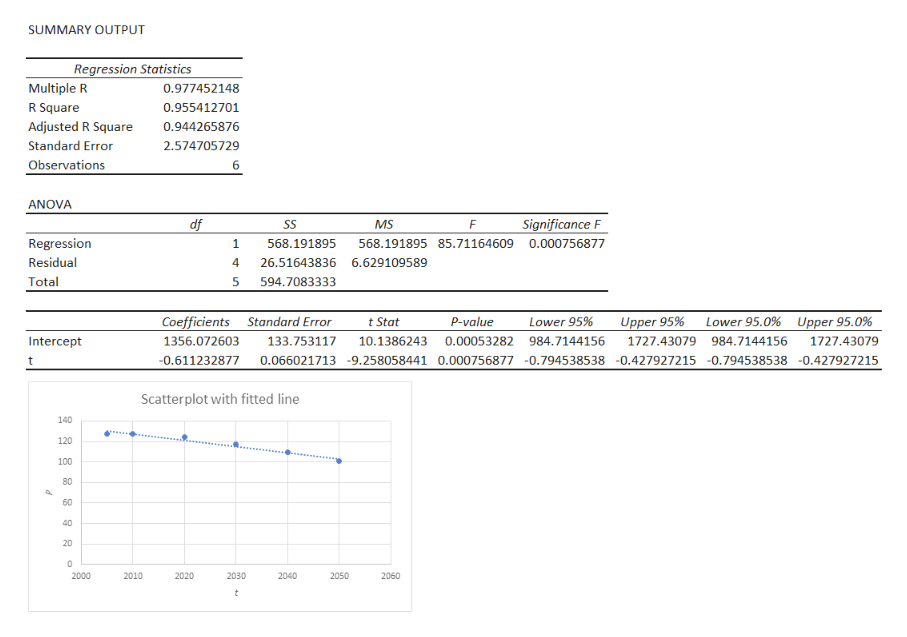The population of a country is expected to drop by 30% over the next 50 years as the percent of its citizens that are elderly increases. Projections for the country's population through 2050 are shown in the table.Year, t200520102020203020402050Population, P (in millions)127.8127.3124.1117.6109.5100.6(b) Use the regression feature of a graphing utility to find a linear model for the data. (Round your numerical values to two decimal places.) P = (c) Identify the slope of the model from part (b). (Round your answer to two decimal places.)(d) Use the linear model to predict the population in 2015, 2035, and 2060. (Round your answers to one decimal place.)Are these predictions reasonable?

Question

The population of a country is expected to drop by 30% over the next 50 years as the percent of its citizens that are elderly increases. Projections for the country's population through 2050 are shown in the table.

 Year, t Population, P (in millions) 2005 2010 2020 2030 2040 2050 127.8 127.3 124.1 117.6 109.5 100.6

(b) Use the regression feature of a graphing utility to find a linear model for the data. (Round your numerical values to two decimal places.)
P =

(c) Identify the slope of the model from part (b). (Round your answer to two decimal places.)

(d) Use the linear model to predict the population in 2015, 2035, and 2060. (Round your answers to one decimal place.)

Are these predictions reasonable?

Step 1

Output:

In Excel, enter the data in cells A1 to A7 (t) and B1 to B7 (P); the first row is for labels.

Go to Data > Data Analysis > Regression.

Enter Input Y Range as \$B\$1:\$B\$7 and Input X Range as \$A\$1:\$A\$7.

Click on the box for Labels.

Click OK.

The regression output will be obtained.

To get the fi...help_outlineImage TranscriptioncloseSUMMARY OUTPUT Regression Statistics Multiple R 0.977452148 R Square 0.955412701 Adjusted R Square 0.944265876 2.574705729 Standard Error Observations 6 ANOVA Significance F df SS MS F Regression 1 568.191895 568.191895 85.71164609 0.000756877 Residual 4 26.51643836 6.629109589 Total 5 594.7083333 Lower 95.0%Upper 95.0% Coefficients 1356.072603 Standard Error t Stat P-value Lower 95% Upper 95% 133.753117 10.1386243 0.00053282 984.7144156 1727.43079 984.7144156 1727.43079 Intercept 0.066021713-9.258058441 0.000756877 -0.794538538 -0.427927215 -0.794538538 -0.4279272 15 0.611232877 t Scatterplot with fitted line 140 120 100 80 60 40 20 C 2000 2010 2020 2030 2040 2050 2060 t fullscreen

Want to see the full answer?

See Solution

Want to see this answer and more?

Our solutions are written by experts, many with advanced degrees, and available 24/7

See Solution
Tagged in

Other